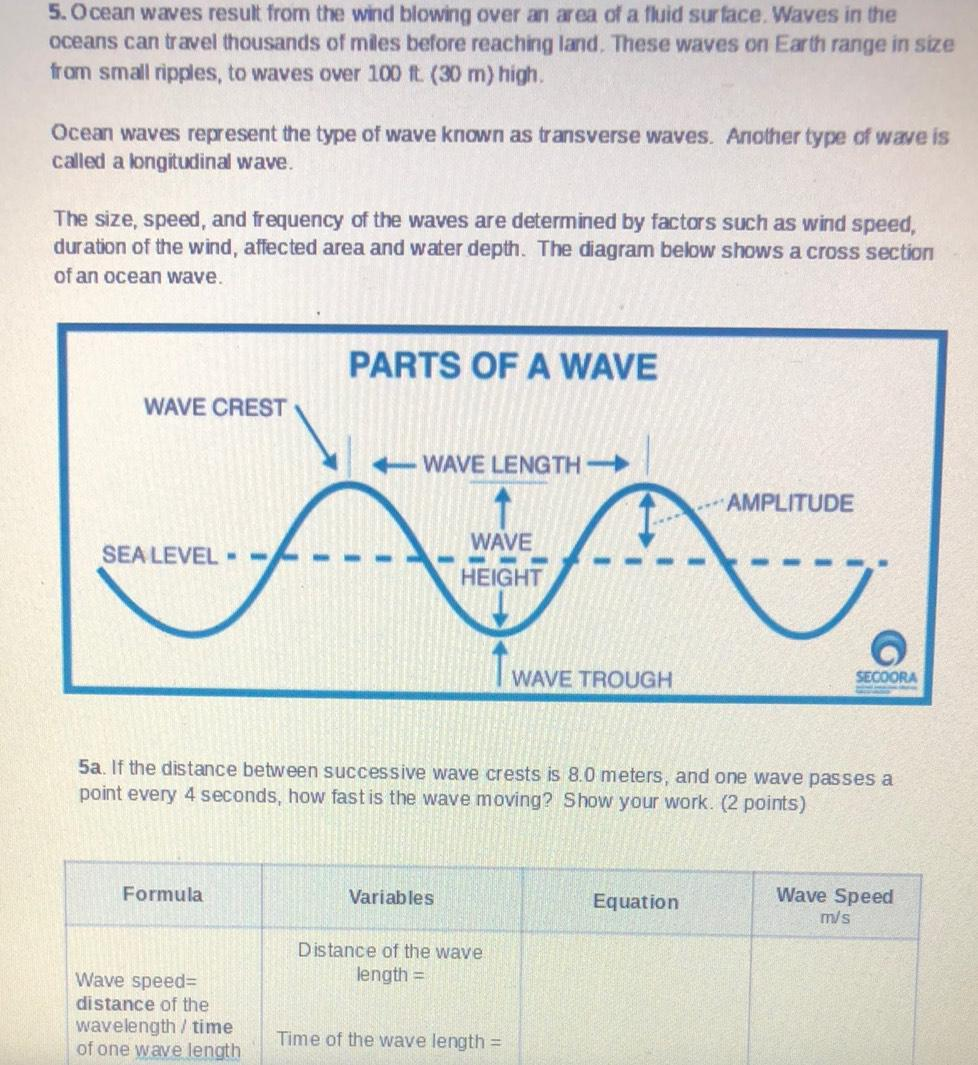Question:

# 5. Ocean waves result from the wind blowing over an area of a fluid surface. Waves in the oceans can travel thousands of miles b5. Ocean waves result from the wind blowing over an area of a fluid surface. Waves in the oceans can travel thousands of miles before reaching land. These waves on Earth range in size from small ripples, to waves over 100 ft. (30 m) high, Ocean waves represent the type of wave known as transverse waves. Another type of wave is called a longitudinal wave. The size, speed, and frequency of the waves are determined by factors such as wind speed, duration of the wind, affected area and water depth. The diagram below shows a cross section of an ocean wave. PARTS OF A WAVE WAVE CREST WAVE LENGTH - AMPLITUDE WAVE SEA LEVEL - HEIGHT T WAVE TROUGH SECOORA 5a. If the distance between successive wave crests is 8.0 meters, and one wave passes a point every 4 seconds, how fast is the wave moving? Show your work. (2 points) Formula Variables Equation Wave Speed m/s Distance of the wave length = Wave speed= distance of the wavelength/time of one wave length Time of the wave length =